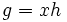Left cosets are in bijection via left multiplication

This article gives the statement, and possibly proof, of a basic fact in group theory.
View a complete list of basic facts in group theory
VIEW FACTS USING THIS: directly | directly or indirectly, upto two steps | directly or indirectly, upto three steps|

Statement

Statement with symbols

Let$H$ be a subgroup of a group$G$ and let$xH$ and$yH$ be two left cosets of$H$. Then, there is a bijection between$xH$ and$yH$ as subsets of$G$, given by the left multiplication by$yx^{-1}$.

Related facts

Related facts about other algebraic structures

• Left cosets of a subgroup are not in bijection via left multiplication in a monoid: If$G$ is a monoid and$H$ is a subgroup of$G$, it is not necessarily true that the left cosets of$H$ are in bijection via left multiplication. In other words, the failure of inverses for elements outside the subgroup can hurt.
• Translates of any subset of a group are in bijection with the subset: For any subset$S$ of a group$G$, the subsets$gS, g \in G$, are all in bijection with$S$. However, they need not be disjoint.
• Left cosets of a subloop are in bijection with the subloop: If$H$ is a subloop of an algebra loop$G$, the left cosets of$H$, i.e., the sets of the form$gH, g \in G$, are all in bijection with$H$. However, they need not be pairwise disjoint.

Significance

In the particular case where the subgroup is also normal, the cosets of the subgroup are congruence classes for the congruence corresponding to the normal subgroup. The result then tells us that all the congruence classes have equal size for any congruence, which tells us that the variety of groups is a congruence-uniform variety (viz, every congruence on it is a uniform congruence). Further information: variety of groups is congruence-uniform

Facts used

1. Invertible implies cancellative in monoid: In particular, we can cancel elements in a group: if$ab = ac$, then$b = c$.

Proof

Given: A group$G$, a subgroup$H$, and two left cosets$xH$,$yH$ of$H$

To prove: Left multiplication by$yx^{-1}$ establishes a bijection between$xH$ and$yH$.

Proof: We prove that left multiplication by$yx^{-1}$ sends$xH$ to$yH$, is surjective, and is injective.

1. Well-defined as a map from$xH$ to$yH$: First, note that if$g = xh$ then$yx^{-1}g = yh$. Thus, any element in$xH$ gets mapped to an element in$yH$.
2. Surjective: Every element of the form$yh$ with$h$ in$H$ arises as$yx^{-1}(xh)$, hence, it arises as the image of left multiplication by$yx^{-1}$. Thus, the map from$xH$ to$yH$ is surjective.
3. Injective: Given two distinct elements$xh_1, xh_2 \in xH$, the elements$(yx^{-1})xh_1 = yh_1$ and$(yx^{-1})xh_2 = yh_2$ are also distinct, because by fact (1), if they were equal, then canceling$yx^{-1}$ from both sides would give$xh_1 = xh_2$. Thus, left multiplication by$yx^{-1}$ sends distinct elements to distinct elements, so the map is injective.

Thus, left multiplication by$yx^{-1}$ is a bijection from$xH$ to$yH$.

Sidenote

In general, there is no natural bijection between two left cosets -- the bijection depends on a choice of element in both cosets (the elements$x$ and$y$ in the above description).